Updating search results...

# 18 Results

View
Selected filters:
• numerical-expressions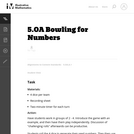Unrestricted Use
CC BY
Rating
0.0 stars

The purpose of this game is to help students think flexibly about numbers and operations and to record multiple operations using proper notation.

Subject:
Mathematics
Numbers and Operations
Material Type:
Activity/Lab
Provider:
Illustrative Mathematics
Provider Set:
Illustrative Mathematics
Author:
Illustrative Mathematics
11/26/2012Unrestricted Use
CC BY
Rating
0.0 stars

The purpose of this task is to generate a classroom discussion that helps students synthesize what they have learned about multiplication in previous grades.

Subject:
Mathematics
Numbers and Operations
Material Type:
Activity/Lab
Provider:
Illustrative Mathematics
Provider Set:
Illustrative Mathematics
Author:
Illustrative Mathematics
05/13/2012Conditional Remix & Share Permitted
CC BY-NC
Rating
0.0 stars

Students are introduced to classroom routines and expectations, and complete a full mathematics lesson. The class discusses how to clearly present work to classmates.&nbsp;Key ConceptsStudents match a numerical expression to its corresponding description in words. Students interpret parentheses and brackets in numerical expressions and they construct viable arguments and critique the reasoning of others. Students learn to use the exponent 2 to represent squaring.Goals and Learning ObjectivesDescribe the classroom routines and expectations.Collaborate with a partner.Critique a partner&rsquo;s reasoning.Connect a numerical expression to its corresponding word description.Learn to use an exponent of 2 to represent squaring.

Subject:
Algebra
Material Type:
Lesson Plan
Author:
Angela Vanderbloom
08/08/2018Conditional Remix & Share Permitted
CC BY-NC
Rating
0.0 stars
Rating
0.0 stars
Subject:
Mathematics
Material Type:
Full Course
Provider:
Pearson
10/06/2016Conditional Remix & Share Permitted
CC BY-NC
Rating
0.0 stars

Expressions

Type of Unit: Concept

Prior Knowledge

Students should be able to:

Write and evaluate simple expressions that record calculations with numbers.
Use parentheses, brackets, or braces in numerical expressions and evaluate expressions with these symbols.
Interpret numerical expressions without evaluating them.

Lesson Flow

Students learn to write and evaluate numerical expressions involving the four basic arithmetic operations and whole-number exponents. In specific contexts, they create and interpret numerical expressions and evaluate them. Then students move on to algebraic expressions, in which letters stand for numbers. In specific contexts, students simplify algebraic expressions and evaluate them for given values of the variables. Students learn about and use the vocabulary of algebraic expressions. Then they identify equivalent expressions and apply properties of operations, such as the distributive property, to generate equivalent expressions. Finally, students use geometric models to explore greatest common factors and least common multiples.

Subject:
Algebra
Mathematics
Material Type:
Unit of Study
Provider:
PearsonConditional Remix & Share Permitted
CC BY-NC
Rating
0.0 stars

Getting Started

Type of Unit: Introduction

Prior Knowledge

Students should be able to:

Solve and write numerical equations for whole number addition, subtraction, multiplication, and division problems.
Use parentheses to evaluate numerical expressions.
Identify and use the properties of operations.

Lesson Flow

In this unit, students are introduced to the rituals and routines that build a successful classroom math community and they are introduced to the basic features of the digital course that they will use throughout the year.

An introductory card sort activity matches students with their partner for the week. Then over the course of the week, students learn about the lesson routines: Opening, Work Time, Ways of Thinking, Apply the Learning, Summary of the Math, and Reflection. Students learn how to present their work to the class, the importance of taking responsibility for their own learning, and how to effectively participate in the classroom math community.

Students then work on Gallery problems to further explore the program’s technology resources and tools and learn how to organize their work.

The mathematical work of the unit focuses on numerical expressions, including card sort activities in which students identify equivalent expressions and match an expression card to a word card that describes its meaning. Students use the properties of operations to identify equivalent expressions and to find unknown values in equations.

Subject:
Mathematics
Material Type:
Unit of Study
Provider:
PearsonConditional Remix & Share Permitted
CC BY-NC
Rating
0.0 stars

Students participate in an icebreaker activity, finding a classmate whose card contains an expression equivalent to the expression on their own card. The resulting student pairs will be partners for this unit. Students spend time exploring the digital course. They learn new symbols for multiplication and detect possible errors in evaluating numeric expressions. The class discusses and decides upon norms for math class.Key ConceptsStudents evaluate numerical expressions and identify equivalent expressions. They explore why the order of operations affects calculation results and how to use parentheses to clearly describe the order of the operations.Goals and Learning ObjectivesEvaluate numerical expressions.Understand the reason for the order of operations and how to use parentheses in numerical expressions.Use the basic features of the application.Create and understand the classroom norms.Use mathematical reasoning to justify an answer.PreparationPrint out the Expressions Icebreaker cards. Select the number of pairs of Partner 1 and Partner 2 cards needed for your class. Shuffle the cards before distributing to students.Write on the board or chart paper: Find a classmate whose card has an expression that is equivalent to the expression on your card.Choose a hand signal or phrase for common activities, such as putting technology away and focusing on the teacher.

Subject:
Algebra
Material Type:
Lesson Plan
09/21/2015Conditional Remix & Share Permitted
CC BY-NC-SA
Rating
0.0 stars

Students are introduced to classroom routines and expectations, and complete a full mathematics lesson. The class discusses how to clearly present work to classmates. Partner work is modeled, and partners then work to match numerical expressions to corresponding word descriptions. Students read and discuss a summary of the math in the lesson, and then write a reflection about their thoughts.Key ConceptsStudents match a numerical expression to its corresponding description in words. Students interpret parentheses and brackets in numerical expressions and they construct viable arguments and critique the reasoning of others. Students learn to use the exponent 2 to represent squaring.Goals and Learning ObjectivesDescribe the classroom routines and expectations.Consider how to present work clearly to classmates.Collaborate with a partner.Critique a partner&rsquo;s reasoning.Connect a numerical expression to its corresponding word description.Learn to use an exponent of 2 to represent squaring.

Subject:
Algebra
Material Type:
Lesson Plan
Author:
Benjamin White
08/23/2018Conditional Remix & Share Permitted
CC BY-NC
Rating
0.0 stars

Students are introduced to classroom routines and expectations, and complete a full mathematics lesson. The class discusses how to clearly present work to classmates. Partner work is modeled, and partners then work to match numerical expressions to corresponding word descriptions. Students read and discuss a summary of the math in the lesson, and then write a reflection about their thoughts.Key ConceptsStudents match a numerical expression to its corresponding description in words. Students interpret parentheses and brackets in numerical expressions and they construct viable arguments and critique the reasoning of others. Students learn to use the exponent 2 to represent squaring.Goals and Learning ObjectivesDescribe the classroom routines and expectations.Consider how to present work clearly to classmates.Collaborate with a partner.Critique a partner&rsquo;s reasoning.Connect a numerical expression to its corresponding word description.Learn to use an exponent of 2 to represent squaring.

Subject:
Algebra
Material Type:
Lesson Plan
09/21/2015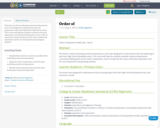Unrestricted Use
CC BY
Rating
0.0 stars

This lesson is about evaluating numerical expressions, and it was designed for adult learners who are preparing to take their High School Equivalency tests. This course will help the students evaluate numerical expressions correctly by following the correct order of operations, which includes the four basic arithmetical operations and the use of exponents and grouping symbols.

Subject:
Mathematics
Material Type:
Lesson
08/08/2019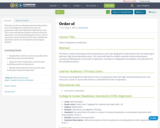Unrestricted Use
CC BY
Rating
0.0 stars

This lesson is about evaluating numerical expressions, and it was designed for adult learners who are preparing to take their High School Equivalency tests. This course will help the students evaluate numerical expressions correctly by following the correct order of operations, which includes the four basic arithmetical operations and the use of exponents and grouping symbols.

Subject:
Mathematics
Material Type:
Lesson
08/08/2019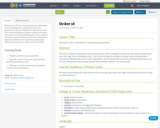Unrestricted Use
CC BY
Rating
0.0 stars

This lesson is about evaluating numerical expressions, and it was designed for adult learners who are preparing to take their High School Equivalency tests. This course will help the students evaluate numerical expressions correctly by following the correct order of operations, which includes the four basic arithmetical operations and the use of exponents and grouping symbols (parentheses, brackets, and curly braces).

Subject:
Mathematics
Material Type:
Lesson
08/08/2019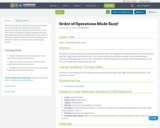Unrestricted Use
CC BY
Rating
0.0 stars

This lesson is about evaluating numerical expressions, and it was designed for adult learners who are preparing to take their High School Equivalency tests. This course will help the students evaluate numerical expressions correctly by following the correct order of operations, which includes the four basic arithmetical operations and the use of exponents and grouping symbols.

Subject:
Mathematics
Material Type:
Lesson
01/17/2017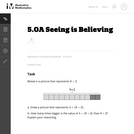Unrestricted Use
CC BY
Rating
0.0 stars

The purpose of this task is to help students see that 4_(9+2) is four times as big as (9+2). Though this task may seem very simple, it provides students and teachers with a very useful visual for interpreting an expression without evaluating it.

Subject:
Mathematics
Numbers and Operations
Material Type:
Activity/Lab
Provider:
Illustrative Mathematics
Provider Set:
Illustrative Mathematics
Author:
Illustrative Mathematics
12/31/2012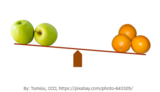Conditional Remix & Share Permitted
CC BY-NC
Rating
0.0 stars

In this seminar, you will learn about variables and how they are used in mathematical expressions. You will also learn what it means to be a like term and how to combine like terms. &nbsp;You will pull variables, expressions, and like terms together so that you can apply them moving forward.StandardsCC.2.2.HS.D.1Interpret the structure of expressions to represent a quantity in terms of its context.CC.2.2.HS.D.2Write expressions in equivalent forms to solve problems.

Subject:
Algebra
Material Type:
Lesson Plan
Author:
Tracy Rains
03/14/2018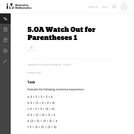Unrestricted Use
CC BY
Rating
0.0 stars

This problem asks the student to evaluate six numerical expressions that contain the same integers and operations yet have differing results due to placement of parentheses. It helps students see the purpose of using parentheses.

Subject:
Mathematics
Numbers and Operations
Material Type:
Activity/Lab
Provider:
Illustrative Mathematics
Provider Set:
Illustrative Mathematics
Author:
Illustrative Mathematics
05/01/2012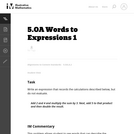Unrestricted Use
CC BY
Rating
0.0 stars

This problem allows students to see words that can describe the expression. Additionally , the words (add, sum) and (product, multiply) are all strategically used so that the student can see that these words have related meanings.

Subject:
Mathematics
Numbers and Operations
Material Type:
Activity/Lab
Provider:
Illustrative Mathematics
Provider Set:
Illustrative Mathematics
Author:
Illustrative Mathematics